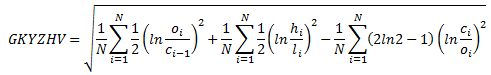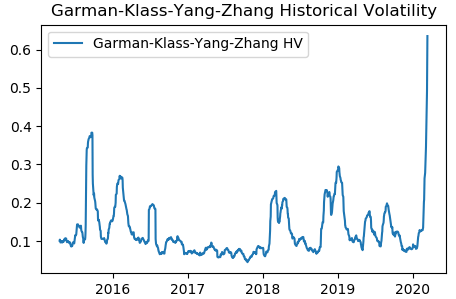Entering text into the input field will update the search result below

# Garman-Klass-Yang-Zhang Historical Volatility Calculation – Volatility Analysis In Python

Jul. 15, 2020 10:46 AM ETSPDR® S&P 500 ETF Trust (SPY)
Please Note: Blog posts are not selected, edited or screened by Seeking Alpha editors.

Seeking Alpha Analyst Since 2013

http://blog.harbourfronts.com

In the previous post, we introduced the Garman-Klass volatility estimator that takes into account the high, low, open, and closing prices of a stock. In this installment, we present an extension of the Garman-Klass volatility estimator that also takes into consideration overnight jumps.

Garman-Klass-Yang-Zhang (GKYZ) volatility estimator consists of using the returns of open, high, low, and closing prices in its calculation. It also uses the previous day’s closing price. It is calculated as follows,where hi denotes the daily high price, li is the daily low price, ci is the daily closing price and oi is the daily opening price of the stock at day i.

We implemented the above equation in Python. We downloaded SPY data from Yahoo finance and calculated the GKYZ historical volatility using the Python program. The picture below shows the GKYZ historical volatility of SPY from March 2015 to March 2020.We note that the GKYZ volatility estimator takes into account overnight jumps but not the trend, i.e. it assumes that the underlying asset follows a GBM process with zero drift. Therefore the GKYZ volatility estimator tends to overestimate the volatility when the drift is different from zero. However, for a GBM process, this estimator is eight times more efficient than the close-to-close volatility estimator.